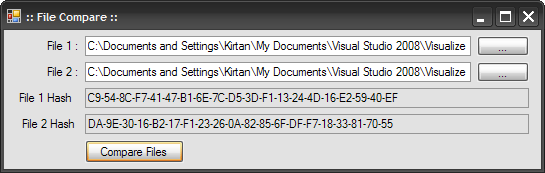# Compare two files with Hash Algorithm

Introduction

This article shows you how to compare two files using HashAlgorithm.We can compare files in many ways like byte by byte comparison. But in some cases comparing through hash algorithm is more efficient method. Hash is actually 20 byte code that is computed by Hash algorithm. If we change even 1 bit in the files the hash code calculated after the changes is totally different. Hence we can immediately know that file content is different.

Technology

Csharp 2.0/3.5

Implementation

Let's implement the hash comparison in our code. Here I am showing what we are trying to makeWe will take two files from the user and then compute their hash code and compare them to see weather they are same? If hash code is same means files are exactly same else they are different.

We need to import two namespace here

using
System.Security.Cryptography;
using System.IO;

I will show you code first and will explain you about code.

private
void btnCompare_Click(object sender, EventArgs e)
{
if(txtFile1.Text != "" && txtFile2.Text !="")
{
HashAlgorithm ha = HashAlgorithm.Create();
FileStream f1 = new FileStream(txtFile1.Text, FileMode.Open);
FileStream f2 = new FileStream(txtFile2.Text, FileMode.Open);
/* Calculate Hash */
byte[] hash1 = ha.ComputeHash(f1);
byte[] hash2 = ha.ComputeHash(f2);
f1.Close();
f2.Close();
/* Show Hash in TextBoxes */
txtHash1.Text = BitConverter.ToString(hash1);
txtHash2.Text = BitConverter.ToString(hash2);
/* Compare the hash and Show Message box */
if (txtHash1.Text == txtHash2.Text)
{
MessageBox.Show("Files are Equal !");
}
else
{
MessageBox.Show("Files are Diffrent !");
}
}
}
private void btnSelectFile1_Click(object sender, EventArgs e)
{
OpenFileDialog ofd = new OpenFileDialog();
if (ofd.ShowDialog() == DialogResult.OK)
{
txtFile1.Text = ofd.FileName;
}
}
private void btnSelectFile2_Click(object sender, EventArgs e)
{
OpenFileDialog ofd = new OpenFileDialog();
if (ofd.ShowDialog() == DialogResult.OK)
{
txtFile2.Text = ofd.FileName;
}
}

In this application we have taken four TextBoxes, 2 for showing Filenames we are comparing and two for showing hash computed for that two files and one compare button.

We are taking files path by btnSelectFile1 and btnSelectFile2 buttons's click event by opening OpenFileDialog and set selected path in txtFile1 and txtFile2.

Now main part is our compare button that contains the focus of our example.

In first line, I have created instance of the HashAlgorithm by

HashAlgorithm
ha = HashAlgorithm.Create();

After that I have opened both user selected files in read mode.

FileStream
f1 = new FileStream(txtFile1.Text, FileMode.Open);
FileStream f2 = new FileStream(txtFile2.Text, FileMode.Open);

We are using ComputeHash() method of the HashAlgorithm class to compute the hash code of the file which will return byte[] array to us.

byte
[] hash1 = ha.ComputeHash(f1);
byte[] hash2 = ha.ComputeHash(f2);

We can not directly show bytes in textBox so we converted byte[] to String using BitConverter class ToString method and shown the computed hash in the TextBox.

Now finally compare hash string in both the textboxes to see whether they are exact or not...

Conclusion

In this article we have seen how to compare two files using Hash Algorithm.